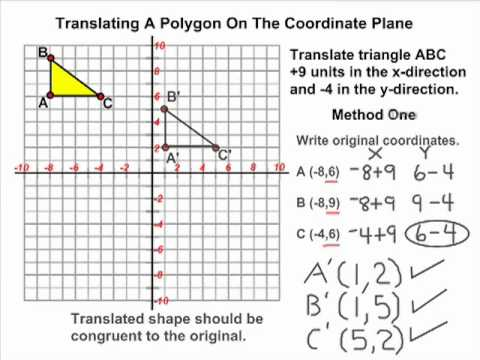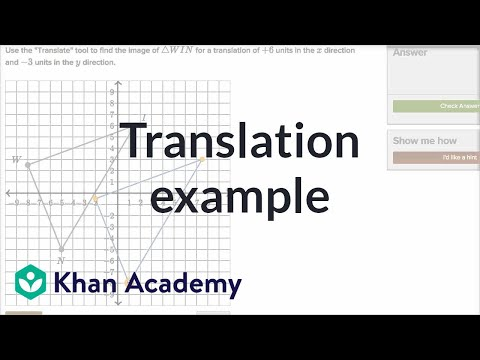## Translation

Subject: Optional Mathematics

#### Overview

A translation is the displacement of a geometrical figure so that its position relative to fixed axes is changed, but not its orientation, size and shape. In translation, every point in the object is moved in the same direction and the same distance. Similarly, the directed line segment which is used for translating an object is called the translation vector.

##### Translation

The concept of translation can be compared with displacement which is a movement through a definite distance in a definite direction. In other words, it is the displacement of a geometrical figure so that only its position relative to fixed axes is changed, but not its orientation, size and shape.

A translation is defined as a correspondence between points and their images points such that each image point is in the same direction from the respective original points.

In translation, every point in the object is moved in the same direction and the same distance.

Let ABCD be a triangle. Let's shift the vertex A to A', B to B' and C to C' in such a way that AA' = BB' = CC' and AA' // BB' // CC'. Then the triangle A'B'C' so obtained is the image of $\triangle$ABC by a translation. Here, each point of $\triangle$ABC has translation an equal distance in the same direction. Corresponding to each point on $\triangle$ABC, there is a unique point on $\triangle$A'B'C'. So, translation is a kind of function.

Again, each side of $\triangle$ABC has translated to an equal and parallel line segment. So, $\triangle$ABC and its image $\triangle$A'B'C' are congruent. It shows that translation preserves congruency and so it is an isometric transformation.

Since a translation is defined in terms of magnitude and direction of displacement, it can be represent by a direction by a directed line segment. In the above example, if $\overrightarrow {AA'}$ = $\overrightarrow {BB'}$ = $\overrightarrow {CC'}$ = $\overrightarrow a$, then $\overrightarrow a$ represent the translation which translated $\triangle$ABC to $\triangle$A'B'C'. The length of directed line segment (i.e. magnitude of $\overrightarrow a$) is the magnitude of displacement and the direction of arrow represents the direction of displacement. Since a directed line segment is a vector, the directed line segment which is used for translating an object is called the translation vector.

Again,

Study the following example:

In this figure, $\triangle$A'B'C' is the image of $\triangle$ABC under reflection in the axis PQ, $\triangle$A"B"C" is the image of $\triangle$A'B'C' under reflection in the axis RS and PQ is parallel to RS.

Here,

AP = PA' and A'R = RA"

BP' = P'B' and B'R' = R'B"

CQ' = Q'C' and C'S' = S'C"

Now,

\begin{align*} AA" &= AP + PA' + A'R + RA' '\\ &= PA' + PA' + A'R + A'R\\ &= 2PA' + 2A'R\\ &= 2 (PA' + A'R)\\ &= 2 PR\\ &= 2 × distance\, between\, PQ\, and\, RS\\ \end{align*}

Similarly,

BB" = 2 × distance between PQ and RS

CC" = 2 × distance between PQ and RS

Here, AA" = BB" = CC' and AA' // BB" // CC". So, by the definition of translation, $\triangle$A"B"C" is image of $\triangle$ABC under a translation. Magnitude of the displacement is 2× distance between parallel axes and direction of displacement is perpendicular the parallel axes from object to image.

This example gives the relationship between translation and reflection.It states that a translation can be expressed as a combination of reflections in two parallel lines.

A combination of reflections in two parallel lines m and n is a translation through twice the distance from m to n.

#### Use of Co-ordinates in Translation

Let the co-ordinates of a point A be (a, b). Then position vector of A is $\overrightarrow {OA}$ = $\begin{pmatrix}a\\ b\\ \end{pmatrix}$.

If this directed line segment $\overrightarrow {OA}$ is used to translate a point P(x, y), it shifts the point P by 'a' units in the horizontal direction(in X- direction) and then 'b' units in the vertical direction (in Y- direction) to reach the point P'(x', y').

Then,

x' = x + a and y' = y + b

These equations are called equations of translation. If the directed line segment $\overrightarrow {OA}$ is denoted by T, then a and b are called X- component and Y- component of T and we write, T = $\begin{pmatrix}a\\ b\\ \end{pmatrix}$.

Here,

T is called a translation vector and it translates any point P(x, y) to the point P' as follows:

T : P (x, y)→ P' (x + a, y + b)

#### Properties of Translation

1. Under a translation, each point of a geometric figure associates a definite image point at the same distance in the same direction.
2. Parallelism is invariant in translation. It means if a translation translates a line segment PQ to P'Q', then slopes of PQ and P'Q' are same i.e. PQ and P'Q' are parallel.
3. Object and image are congruent in translation.
4. Lines joining the corresponding points of the object and its image are equal and parallel.
##### Things to remember
• A translation is defined as a correspondence between points.
• In translation, every point in the object is moved in the same direction and the same distance.
• The directed line segment which is used for translating an object is called the translation vector.
• Under a translation, each point of a geometric figure associates a definite image point at the same distance in the same direction.
• The lines joining the corresponding points of the object and its image are equal and parallel.
• It includes every relationship which established among the people.
• There can be more than one community in a society. Community smaller than society.
• It is a network of social relationships which cannot see or touched.
• common interests and common objectives are not necessary for society.
##### Videos for Translation##### Transformations - Translating A Triangle On The Coordinate Plane##### Translation example | Transformations | Geometry | Khan Academy

Soln:

Construction procedure:

(i) Draw AA' from the point A which is parallel and equal to $\overrightarrow{P}$.

(ii) Draw BB' from the point B which is parallel and equal to$\overrightarrow{P}$.

(iii) Also, draw CC' from the point C which is parallel and equal to$\overrightarrow{P}$.

Then join A', B' and C'. So that the triangle A'B'C' is the image of triangle ABC which is shown in the figure by shaded portion.

Soln:

Construction procedure:

As shown in the above figure, EE' is drawn from the point E which is parallel and equal to$\overrightarrow{P}$. Similarly, FF' and DD' are drawn from the points F and D which are also equal and parallel to$\overrightarrow{P}$. Then D', E' and F' are joined so that the triangle D'E'F' is the image of triangle DEF under translation through$\overrightarrow{p}$.

Soln:

Construction procedure:

As shown in the above figure, GG', HH' and II' are drawn from the points G, H and I respectively. which are equal and parallel to$\overrightarrow{P}$. Then by joining G', H' and I' we get the image triangle G'H'T' of the triangle GHI under the translation vector$\overrightarrow{P}$.

Soln:

Construction procedure:

Here, in the figure, AB and DC are parallel and equal to the given vector$\overrightarrow{P}$. So, images of A and D are A' and D' at B and C respectively. Again, CC' and BB' are drawn from the point C and B which are parallel and equal to the vector$\overrightarrow{P}$. Then join A', B', C', and D' so that the parallelogram A"B'C'D' is the image of parallelogram under the translation through the vector$\overrightarrow{P}$.

Soln:

Given translation component =$\begin{pmatrix}4\\7\\ \end{pmatrix}$

A(-1, 1)→ A'(4, -1), 7+1) = A'(3, 8)

∴ T(A) =(3, 8) [ p(a, b)→ P'(a+x, b+y) where T =$\begin{pmatrix}x\\y\\ \end{pmatrix}$ ]

B(-1, -3)→B'(4-1, 7-3) = B'(3, 4)

∴T(B) = (3, 4)

C(-5, 7)→ C'(4-5, 7+7) = C'(-1, 14)

∴T(C) = (-1`, 14)

D(6, 0)→ D'(6+4, 0+7) = D'(10, 7)

∴T(D) = (10, 7)

E(0, 7)→ E'(0+4, 7+7) = E'(4, 14)

∴ T(E) = (4, 14)

F(6, -6)→ F'(4+6, -6 +7) = F'(10, 1)

∴ T(F) = (10, 1)

G(8, 8)→ G'(4+8, 8+7) = G'(12, 15)

∴ T(G) = (12, 15)

H(5, 9)→ H'(5+4, 9+7) = H'(9, 16)

∴T(H) =(9, 16)

I(2, 11)→ I'(2+4, 11+7) = I'(6, 18)

∴ T(I) = (6, 18)

j(-3, -3)→ J'(4-3, 7-3 ) = J'(1, 4)

∴ T(J) = (1, 4)

Soln:

Let, E $\begin{pmatrix}x\\y\\ \end{pmatrix}$ translates the point (5, 7) into (3,9)

Then we have, $\begin{pmatrix}x\\y\\ \end{pmatrix}$ + $\begin{pmatrix}5\\7\\ \end{pmatrix}$ =$\begin{pmatrix}3\\9\\ \end{pmatrix}$

or, $\begin{pmatrix}x\\y\\ \end{pmatrix}$ =$\begin{pmatrix}3\\9\\ \end{pmatrix}$ -$\begin{pmatrix}5\\7\\ \end{pmatrix}$ =$\begin{pmatrix}3-5\\9-7\\ \end{pmatrix}$ =$\begin{pmatrix}-2\\2\\ \end{pmatrix}$

∴ Translation component =$\begin{pmatrix}-2\\2\\ \end{pmatrix}$

Again, translation of P(8, 4) by T =$\begin{pmatrix}-2\\2\\ \end{pmatrix}$ is

∴ P'(y', x') = (8 - 2, 4 + 2) = (6, 6). Ans

Soln:

Given, translation component =$\begin{pmatrix}3\\5\\ \end{pmatrix}$

Then image of triangle OPQ is given as:

O(0, 0)→ O'(3 + 0, 5 + 0) = O'(3, 5)

P(6, 3)→ P'(3 + 6, 5 + 3) = P'(9, 8)

Q(4, 7)→ Q'(3 + 4, 5+7) = Q'(7, 12)

The graph of triangle OPQ and its image triangle O'P'Q' are as through below: Where the image is the shaded region.

Soln:

Let the component$\begin{pmatrix}x\\y\\ \end{pmatrix}$ translates A(-3, -1)→ C(5, 1)

So, $\begin{pmatrix}x\\y\\ \end{pmatrix}$ + $\begin{pmatrix}-3\\-1\\ \end{pmatrix}$ =$\begin{pmatrix}5\\1\\ \end{pmatrix}$

or,$\begin{pmatrix}x-3\\y-1\\ \end{pmatrix}$ =$\begin{pmatrix}5\\1\\ \end{pmatrix}$

or, x - 3 = 5 ∴x = 8

or, y - 1 = 1 ∴y = 2

∴ component of $\overrightarrow{AC}$$\begin{pmatrix}8\\2\\ \end{pmatrix}$. Ans

Alternatively,

translation vector = $\overrightarrow{AC}$

= $\overrightarrow{OC}$-$\overrightarrow{OA}$

=$\begin{pmatrix}5\\1\\ \end{pmatrix}$-$\begin{pmatrix}-3\\-1\\ \end{pmatrix}$

=$\begin{pmatrix}8\\2\\ \end{pmatrix}$

Soln:

We have,$\overrightarrow{AC}$ =$\begin{pmatrix}8\\2\\ \end{pmatrix}$.

Now, ytranslation of parallelogram ABCD by$\begin{pmatrix}8\\2\\ \end{pmatrix}$, we get

A(-3, -1)→ A'(8-3, 2-1) = A'(5, 1)

B(-1, -1)→ B'(8 - 1, 2 - 1) = B'(7, 1)

C(5, 1)→ C'(8 + 5, 2 + 1) = C'(13, 3)

and D(3, 1) → D'(8 + 3, 2 + 1) = D'(11, 3)

The graph of parallelofram ABCD and its image parallelogram A'B'C'D' are as shown in graph where the image is shaded.

Soln:

Here, the translation compponent $\begin{pmatrix}x\\y\\ \end{pmatrix}$ translates A(-3, 0) to D(3, -1). So, we have

$\begin{pmatrix}x\\y\\ \end{pmatrix}$ +$\begin{pmatrix}-3\\0\\ \end{pmatrix}$ = $\begin{pmatrix}3\\-1\\ \end{pmatrix}$

or,$\begin{pmatrix}x-3\\y+0\\ \end{pmatrix}$ =$\begin{pmatrix}3\\-1\\ \end{pmatrix}$

or, x - 3 = 3 ∴ x = 6

and y + 0 = -1 ∴ y = -1

Here, the component vector =($\frac{6}{-1}$) which translate quadrilateral ABCD as follows:

A(-3,0)→A'(6-3,-1+0)=A'(3,-1)

B(-2,2)→B'(6-2,-1+2)=B'(4,1)

C(1,3)→C'(6+1,-1+3)=C'(7,2)

D(3,-1)→D'(6+3,-1-1)=D(9,-2)

∴The graph of quadrilateral ABCD and its image A'B'C'D' are shown below where the shaded region gives the image.

Construction procedure: Here from the points Q,R,S,T,U,V,W,X,Y and Z the lines QQ',RR',TT', UU',VV',WW',XX',YY',and ZZ' are drawn equal and parallel to the given vector $\overrightarrow{P}$.Then the points Q',R',S',T',U',V',W',X',Y', and Z', are joined so that the figure Q'R'S'T'U'V'W'X'Y'Z' is the image of the given figure QRSTUVWXYZ under the translation vector $\overrightarrow{P}$.

Construction procedure:As shown in the above figure from the points E, F,G,H,I,J,K,L,M, and N, lines EE',FF',GG',HH',II',JJ',KK',LL',MM',and NN', are drawn equal and parellel to the vector$\overrightarrow{P}$.Then the points E',F',G',H',I',J',K',L',M' and N' are joined.Then the figure E'F'G'H'I'J'K'L'M'N' is the image of the figure EFGHIJKLMN under the given vector$\overrightarrow{P}$.